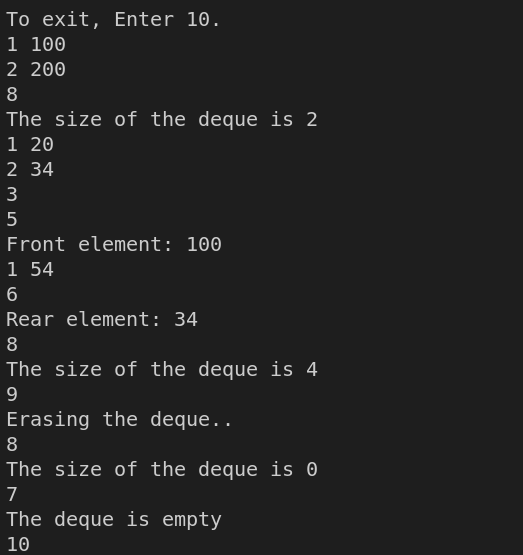# Implementation of Deque Using Doubly Linked List

## Understanding

Deque, or Double Ended Queue, is a generalized Queue data structure that supports insert and delete from both ends. There are various ways to implement a deque, for example, using a circular array. In this blog, we will implement the different functionalities of the Deque data structure using a doubly-linked list.

## Problem Statement

You need to implement a deque data structure that supports the following operations/functionalities -

1. Insert a value at the beginning
2. Insert a value at the end
3. Delete a value from the beginning
4. Delete a value from the end
5. Outputs the value at the beginning
6. Outputs the value at the end
7. Determines if it is empty
8. Outputs its size

## Input Method

To do an operation on the deque data structure, we will use the number assigned to that operation in the problem statement. For example -

i. to insert a value of 100 at the end of a deque, we will provide the following input -

2 100

ii. to output the size of the deque, we should provide the following input -

8

and so on.

1 100

2 200

8

1 20

2 34

3

5

1 54

6

8

## Output

The size of the deque: 2

Front element: 100

Rear element: 34

The size of the deque: 4

## Approach

We will implement using the concept of a doubly linked list. We will maintain two pointers - Front and Rear to keep track of the beginning and end of the queue. When we need to insert or delete elements from the beginning, we will appropriately tweak the Front pointer to make the necessary changes. The same goes with the Rear pointer usage.

We can maintain a variable size to keep track of the size of the data structure. In the case of insert statements, we will increase the size by one, and in the case of delete, we will decrease the size by one. We can use the value of the size variable to determine if the deque is empty or not.

### Algorithm

We will implement each of the functionalities in the form of functions -

1. Create a Node structure to represent a node in the doubly-linked list.
2. Create a class Deque to represent the deque data structure to be implemented.
3. Create front and rear pointers to keep track of the beginning and end of the deque and initialize the size variable with zero.
4. Create the following functions as part of the class Deque.

insertFront

This function takes an integer as an argument. Create a new node in the doubly-linked list with value as the integer provided as the argument. Then execute the following statements -

IF front == NULL, then

rear = front = newNode

ELSE

newNode->next = front

front->prev = newNode

front = newNode

insertEnd

This function takes an integer as an argument. Create a new node in the doubly-linked list with value as the integer provided as the argument. Then execute the following statements -

IF rear == NULL, then

rear = front = newNode

ELSE

newNode->prev = front

rear->next = newNode

rear = newNode

deleteFront

Check if the deque is empty. If yes, return.

Node* temp = front

front = front->next

IF front == NULL

rear = NULL

ELSE

front->prev = NULL

Deallocate space for temp

deleteRear

Check if the deque is empty. If yes, return.

Node* temp = rear

rear = rear->prev

IF rear == NULL

front = NULL

ELSE

rear->next = NULL

Deallocate space for temp

isEmpty

Return if the deque is empty based on the value of the size variable.

getFront

If the queue is empty, output a garbage value. Otherwise, return the value of the node pointed to by the front pointer.

getRear

If the queue is empty, output a garbage value. Otherwise, return the value of the node pointed to by the rear pointer.

### Execution### Time Complexity

The time complexities of the various functions are -

1. insertFront - O(1)
2. insertRear - O(1)
3. deleteFront - O(1)
4. deleteRear - O(1)
5. getFront - O(1)
6. getRear - O(1)
7. isEmpty - O(1)
8. size - O(1)
9. erase - O(n)

### Space Complexity

The space complexity is O(n) to hold the deque.

## Key Takeaways

One of the most significant concepts and data structures to grasp while preparing for interviews is the linked list. Knowing how to use a linked list effectively can be quite beneficial in coding interviews. In this blog, we built a deque data structure using a doubly-linked list. We saw how to handle delete operations in a doubly-linked list using a straightforward algorithm.

There are many more applications of doubly-linked lists. Hence learning never stops, and there is a lot more to learn. So head over to our practice platform CodeStudio to practice top problems, attempt mock tests, read interview experiences, and much more. Till then, Happy Coding!